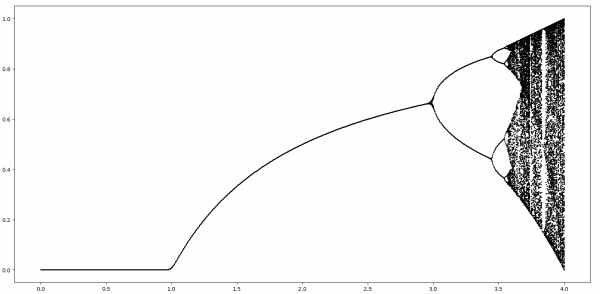# Does chaos imply period 3?

Sharkovskii’s theorem says that if a continuous map f from an interval I to itself has a point with period 3, then it has a point with period 5. And if it has a point with period 5, then it has points with order 7, etc. The theorem has a chain of implications that all begins with 3. If you have points with period 3, you have points of all periods.

Now suppose you want to go “upstream” in Sharkovskii’s chain of implications. If you have a point with period 5, do you have a point with period 3? The answer is no: a map can have points with period 5 without having points of period 3, though it necessarily has points with all other positive integer periods except 3.

There’s an example illustrating the claim above that I’ve seen in multiple places, but I haven’t seen it presented graphically. You could work through the example analytically, but here I present it graphically.

This is the example function written in Python.

```    def f(x):
assert(1 <= x <= 5)

if x < 2:
return 1 + 2*x
if x < 3:
return 7 - x
if x < 4:
return 10 - 2*x
if x <= 5:
return 6 - x
```

Here’s a graphical demonstration that f has a fixed point, but no points of period 3.The only point fixed under applying f three times is the point that was already fixed under applying f once.

This graph shows that f has points with period 5:By Sharkovskii’s theorem f must have points with all other periods, except 3. Here’s a demonstration that it has points with period 6.The map f is chaotic, but it does not have a point with period 3.

## Logistic map

Let’s look at the most famous chaotic map, the logistic map.

f(x) = rx (1 – x)

where x is in [0, 1] and r is in [0. 4].The images above shows orbits as r ranges over [0, 4]. Clearly f has points with period 2. There’s a whole interval of values of r that lead to points with period 2, roughly for r between 3 and 3.5. And we can see for r a little bigger there are points of period 4. But is there any point with period 3?

We can look for points of period 3 at the end of the plot, where r = 4, using Mathematica.

Define

`    f[x_] := 4 x (1 - x)`

and look for points where f³(x) = x using

`    Factor[f[f[f[x]]] - x]`

This shows that the solutions are the roots of

x (-3 + 4 x) (-7 + 56x – 112x² + 64 x³) (-3 + 36x – 96x² + 64x³)

The first two roots are fixed points, points of period 1, but the roots of the two cubic factors are points with period 3.The cubics clearly have all their roots in the interval [0,1] and we could find their numerical values with

`    NSolve[f[f[f[x]]] == x, x]`

Although the roots are fixed points, they are unstable fixed points, as demonstrated at the bottom the next post.